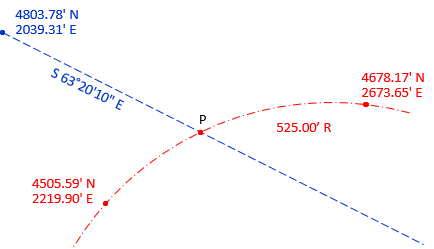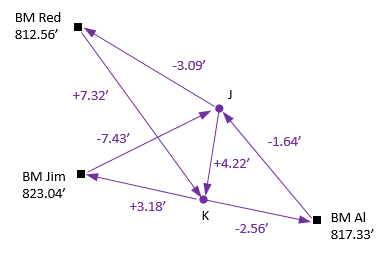### What do the following surveying situations have in common?

#### Situation 1

A +4.0% grade is followed by a -2.0% grade on a vertical alignment. Using the information shown in Figure A-1, what are the station and elevation of the PVI?Figure A-1Vertical Alignment

#### Situation 2

With the information shown in Figure A-2, what are the coordinates at the center lines intersection?Figure A-2Center Lines Intersection

#### Situation 3

Given the elevation differences for the level circuit in Figure A-3, what are the elevations of points J and K?Figure A-3Level Circuit

While they differ considerably, the common thread is each can solved using simultaneous equations. Sometimes only simple math is needed, other times complex math like (shudder) calculus must be applied.

Software has largely taken the place of manual survey calculations. However, unless software can address every type of problem (it can't) sometimes the surveyor must resort to pencil, paper, and calculator.

This Topic covers basic concepts of manual simultaneous equation solution. The emphasis is on unique solutions, that is, there are same number of equations as things being determined. That is one way these three situations differ from each other: only Situation 1 has a unique solution. Situation 2 has two geometric solutions, one which can be eliminated by observation. Situation 3 is a typical situation in surveying when we make more measurements than necessary to determine what we want. For this we normally use a method to compute "best" elevations for the two points. One of these methods is Least Squares, which will be covered in a subsequent Topic.